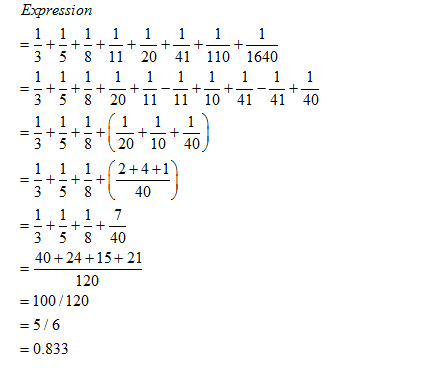• This is an assessment test.
• These tests focus on the basics of Maths and are meant to indicate your preparation level for the subject.
• Kindly take the tests in this series with a pre-defined schedule.

## Basic Maths: Test 22

Congratulations - you have completed Basic Maths: Test 22. You scored %%SCORE%% out of %%TOTAL%%. You correct answer percentage: %%PERCENTAGE%% . Your performance has been rated as %%RATING%%
 Question 1
The value of $\displaystyle \frac{2\frac{1}{3}-1\frac{2}{11}}{5+\frac{1}{3+\frac{1}{3+\frac{1}{3}}}}$ is
 A 43/109 B 38/175 C 1/109 D 116/109
Question 1 Explanation:
We will do this expression by section vise
In first section we will solve for numerator and then for denominator
$\displaystyle \begin{array}{l}\frac{\frac{7}{3}-\frac{13}{11}}{5+\frac{1}{3+\frac{1}{\frac{9+1}{3}}}}\\=\frac{\frac{77-39}{33}}{5+\frac{1}{3+\frac{3}{10}}}\\=\frac{\frac{38}{33}}{5+\frac{1}{\frac{30+3}{10}}}\\=\frac{\frac{38}{33}}{5+\frac{10}{33}}\\=\frac{\frac{38}{33}}{\frac{165+10}{33}}\\=\frac{38}{33}\times \frac{33}{175}\\=\frac{38}{175}\end{array}$
 Question 2
The value of $\displaystyle 5+\frac{5}{3+\frac{1}{3+\frac{1}{3}}}$ is
 A 11/40 B 214/33 C 46/11 D 215/33
Question 2 Explanation:
The given fraction can be solved by
$\displaystyle 5+\frac{5}{3+\frac{1}{\frac{9+1}{3}}}$ $\displaystyle \begin{array}{l}=5+\frac{5}{3+\frac{3}{10}}\\=5+\frac{5}{\frac{30+3}{10}}\\=5+\frac{50}{33}\\=\frac{165+50}{33}\\=\frac{215}{33}\end{array}$
 Question 3
$\displaystyle \frac{1}{3}+\frac{1}{5}+\frac{1}{8}+\frac{1}{11}+\frac{1}{20}+\frac{1}{41}+\frac{1}{110}+\frac{1}{1640}$
 A 0.97 B 0.99 C 5/6 or 0.83341 D 1.23
Question 3 Explanation:Question 4
Each boy contributed rupees equal to the number of girls and each girl contributed rupees equal to the number of boys in a class of 60 students. If the total amount thus collected is Rs.1600, how many boys are there in the class?
 A 30 B 25 C 50 D None
Question 4 Explanation:
If the number of boys = p
Then number of girls
$\displaystyle \begin{array}{l}=60-p\\\therefore \,\,\,\left( 60-p \right)p+\left( 60-p \right)p=1600\\\Rightarrow 2\,\left( 60p-{{p}^{2}} \right)=1600\\\Rightarrow {{p}^{2}}-60p+800=0\\\Rightarrow {{p}^{2}}-40p-20p+800=0\\\Rightarrow p\left( p-40 \right)-20\left( p-40 \right)=0\\\Rightarrow \left( p-20 \right)\left( p-40 \right)=0\\\Rightarrow p=20\,\,\,or\,\,\,40\end{array}$
 Question 5
If the amount in Rs.crores earmarked for Malaria Eradication Programme is 142, AIDs Control Programme is 141, T.B. Control Programme is 80, Leprosy Eradication Programme is 78 and for Control of Blindness is 59; how much total amount in Rs. Crores is earmarked for all the given programmes together?
 A 300 B 400 C 480 D 500
Question 5 Explanation:
$\displaystyle \begin{array}{l}=\left( 142+141+80+78+59 \right)\,\,Rs.\,\,crore.\\=Rs.\,\,500\,crore\end{array}$